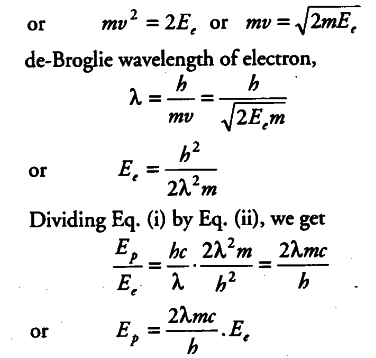# Why are de-Broglie waves associated with a moving football not visible?

Why are de-Broglie waves associated with a moving football not visible? The wavelength A, of a photon and the de-Broglie wavelength of an electron have the same value. Show that the energy of the photon is 2λmc/h times the kinetic energy of the electron , where, m,c and h have their usual meanings.

The magnitude of the wavelength of de-Broglie waves associated with a moving football is extremely
small(λ = h / mv < {{10}^{-34}} m) much less than that of visible region and are therefore not visible.
Wavelength of photon = λ
Energy of photon , { E }_{ p } = hc / λ
Kinetic energy of an electron = , { E }_{ e } =1/2 m {{v}^{2}}